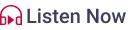# Fundamental Analysis For Mutual Fundsby Nutan Gupta 30/01/2017Fundamental Analysis takes into consideration various elements like the revenue, expenses, and income of the company. It also looks at the growth prospects of the company and management of the company. The fundamental analysis approach is based on the overall macro-economic factors which can have an impact on the stock. Fundamental Analysis is a long-term investment strategy.

There are some fundamental factors one should consider while investing in mutual funds.

## Sector Prospects of the Fund

Every mutual fund has a certain amount of exposure to a particular sector. While investing in a mutual fund scheme, look at the sector in which the fund has the highest exposure to and how that sector is performing. It is not wise to only look at the past performance of the sector. It is very important to analyse what the sector has in store for its investors in the future. Also, one needs to analyse the impact of macro-economic conditions on that sector as a whole.

## Financial Valuation of the Fund

The financial valuation of a mutual fund can be determined by its P/E (price to earnings) ratio. The P/E of a scheme is derived by using a weighted average of underlying stocks. Basically, it is the average of the P/E of all the stocks that are present in a fund’s portfolio.

A higher P/E ratio of the scheme indicates that the stocks in the scheme are valued at a premium. This reflects a growth-based approach of the fund manager. On the other hand, a lower P/E indicates a conservative approach of the fund manager. Here, the fund manager looks for stocks whose stock prices have been beaten down and there is a hope for these prices to rise significantly in the future. Such stocks yield great results over a longer period of time.

## Ratios

Ratios are used to identify the given amount of risk attached to a mutual fund.

### Sharpe Ratio

This ratio is used to calculate the risk-adjusted return. It is calculated as -

Am-Rf/Std

Where, Am is the Arithmetic Mean of the portfolio being evaluated

Rf is the Risk Free Rate

Std is the Standard Deviation of the portfolio being evaluated

### Sortino Ratio

This ratio measures the performance of an investment relative to the downward deviation. It is calculated as -

(R)-Rf/SD

Where, (R) is the expected return

Rf is the risk free rate of return

SD is the standard deviation of the negative asset return